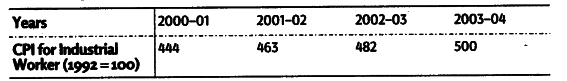# Given the following data,Calculate the inflation rate

Given the following data,Calculate the inflation rate.

Inflation rate for different years is calculated as .

Year 2001-02 =463-444/444x 100 = 4.28 %

Year 2002-03 =482 - 463 /463x 100 = 4.10 %

Year 2003-04 = 500-482/482 x 100= 3.73 %

Consumer Price Index (CPI) of industrial workers declined in all the years.